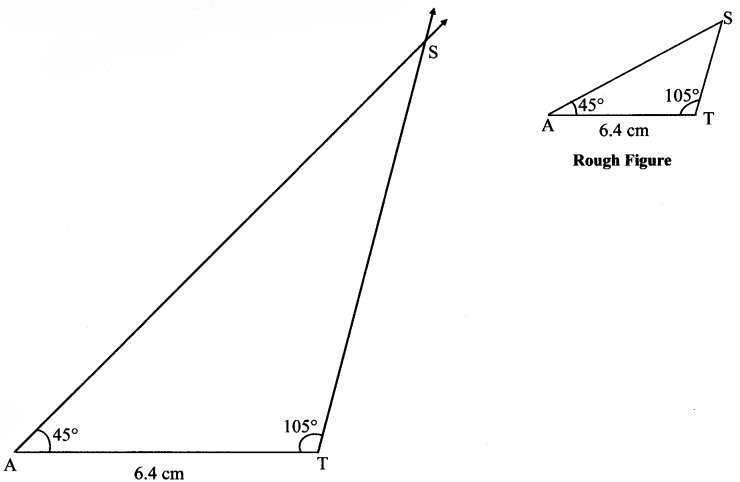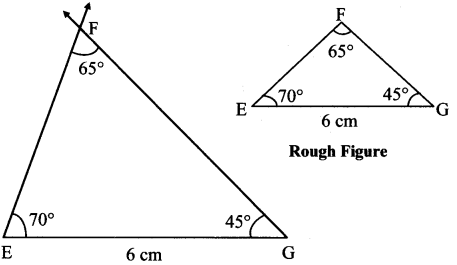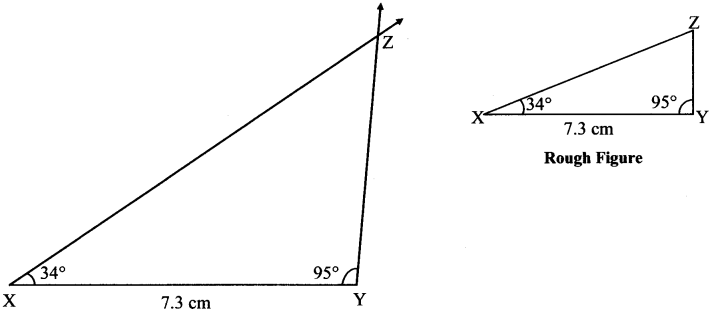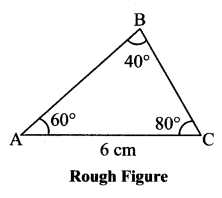# Maharashtra Board Class 7 Maths Solutions Chapter 1 Geometrical Constructions Practice Set 4

## Maharashtra State Board Class 7 Maths Solutions Chapter 1 Geometrical Constructions Practice Set 4

Construct triangles of the measures given below:

Question 1.
In ∆SAT, l(AT) = 6.4 cm, m∠A = 45°, m∠T = 105°.
Solution:Question 2.
In ∆MNP, l(NP) = 5.2 cm, m∠N = 70°, m∠P = 40°
Solution:Question 3.
In ∆EFG, l(EG) = 6 cm, m∠F = 65°, m∠G = 45°.
Solution:
In ∆EFG,
m∠E + m∠F + m∠G = 180° …(sum of measures of angles of a triangle)
m∠E + 65° + 45° = 180°
m∠E + 110° = 180°
m∠E = 180° – 110°
m∠E = 70°Question 4.
In ∆XYZ, l(XY) = 7.3 cm, m∠X = 34°, m∠Y = 95°.
Solution:Maharashtra Board Class 7 Maths Chapter 1 Geometrical Constructions Practice Set 4 Intext Questions and Activities

Question 1.
In ∆ABC, m∠A = 60°, m∠B = 40°, l(AC) = 6 cm. (Textbook pg. no. 5)
1. Can you draw ∆ABC?
2. What further information is required before it can be drawn?
3. Which property can be used to get it?
4. Draw the rough figure to find out.
Solution:
1. ∆ABC cannot be drawn using the given information.
Seg AC is included inside the angles ∠A and ∠C. Since measure of ∠C is not known, the triangle cannot be drawn.
2. To draw the triangle, measure of ∠C is required.
3. The property of sum of the measures of the angles of a triangle can be used to find out m∠C.
4. In ∆ABC,
m∠A + m∠B + m∠C = 180°
∴ 60° + 40° + m∠C = 180°
∴ 100° + m∠C = 180°
m∠C = 180°- 100°
∴ m∠C = 80°